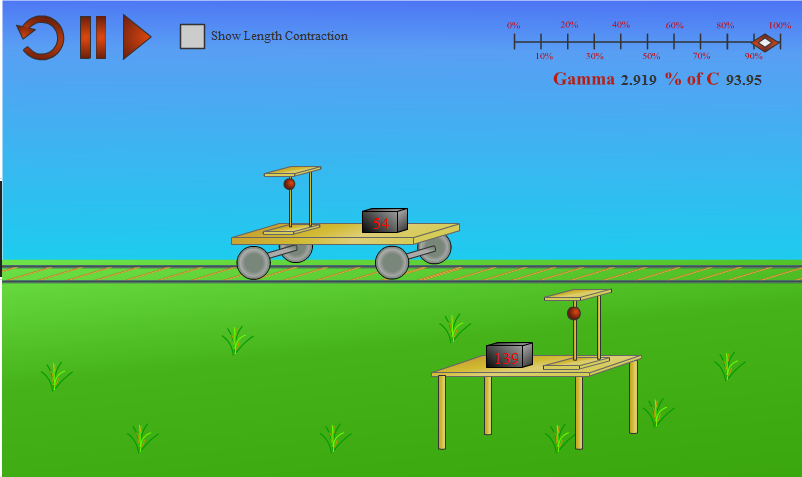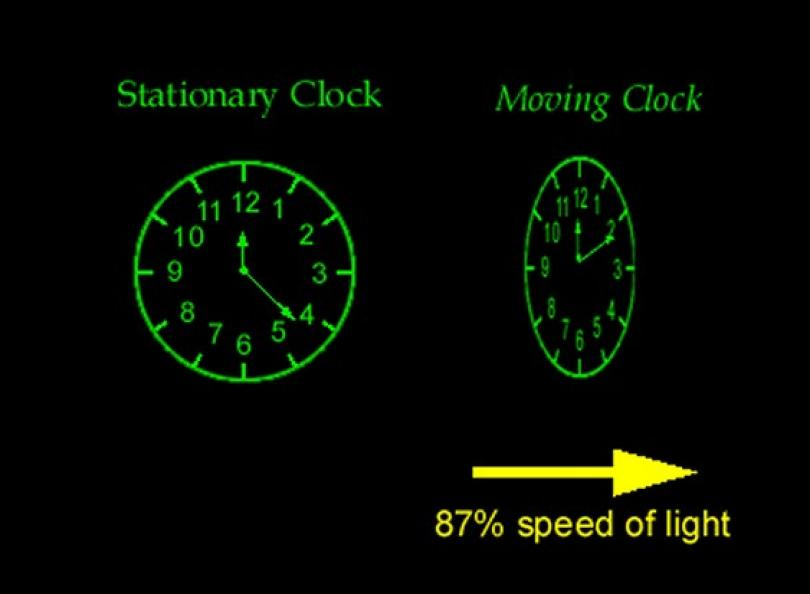# Difference Between Time Dilation and Length Contraction

Length contraction and time dilation are related to the theory of relativity. They are the effects and the end results of the theory. These two effects can easily describe one of the most complicated phenomena in the world of science.

There are two basic differences between length contraction and time dilation;

While time dilation is just an extension or expansion of time from the moving frame, the length contraction is the reduction of length, or its shrinkage.

Secondly, γ (any number between 0 to infinity – a variable ) connects linearly to the time formula, which means it is directly proportional. On the other hand, it connects inversely in the formula of length.

### Instructions

• 1

Length Contraction

This concept is mostly studied under relativity. The special theory of relativity only talks about the inertial frames. The basic concept is that there are no objects which move in the inertial frame and have velocities greater than velocity of light. The term γ, in this case, is always equal to the root of 1/ (1-V2/C2). When V tends to C, γ reaches infinity.

On the other hand, it is equal to 1 when V is smaller than C. The length contraction, however, is derived from the Lorentz equation of transformation. The length one an object is the length which is measured in one frame. The improper length, in this theory, must be either smaller or equal to the actual length.

Hence the formula Improper Length = Proper Length / γ gives the perfect shape to the theory as a whole.

- Image courtesy: ibphysicsstuff.wikidot.com• 2

Time Dilation

Defining time properly is quite difficult. However, scientists believe that proper time is that time which is measured by an observer who is not moving at the speed of the event itself.

On the other hand, the improper time is its opposite.

If the observer is moving at a velocity of V, which is relative to the velocity of the event, then the time recorded by that observer is improper time.

The Lorentz equation has proven that the time recorded in the event frame is always smaller, or equal, to the time which is recorded by the moving frame.

This relationship and difference between improper time and proper time can be perfectly explained with the help of the following equation:

improper time interval = γ x proper time interval.

This relationship becomes a classical relationship when γ becomes 1 after velocity is almost equal to zero as compared to C.

- Image courtesy: zibango.wordpress.com•